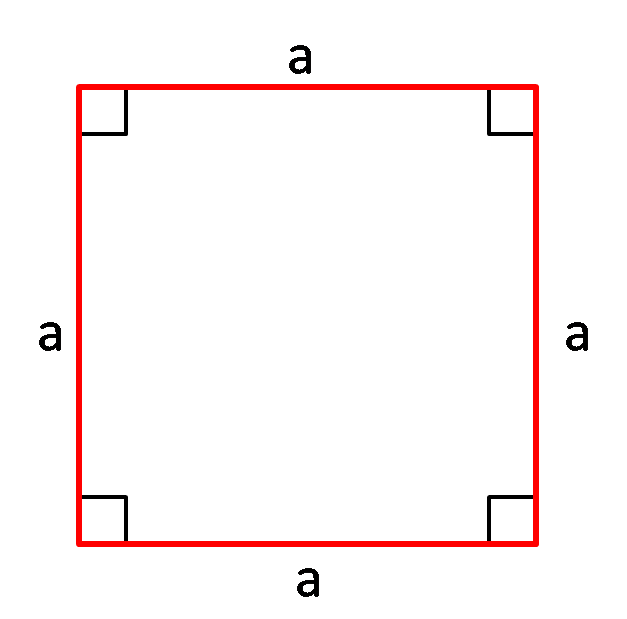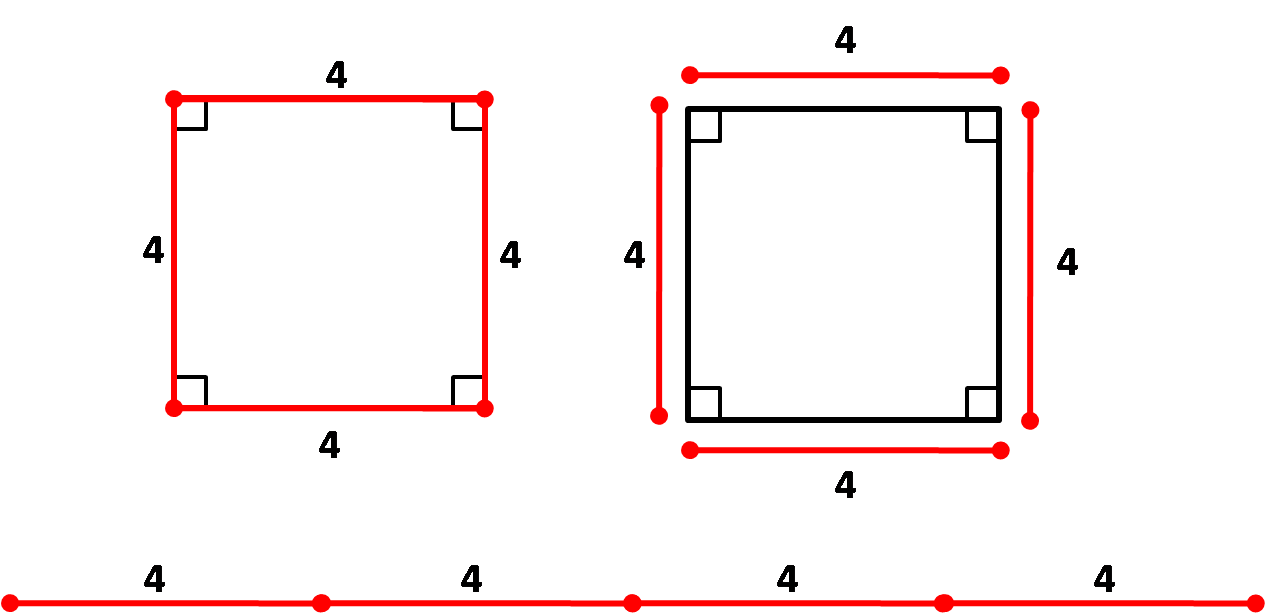# Perimeter of a Square Calculator

Created by Hanna Pamuła, PhD
Reviewed by Bogna Szyk and Adena Benn
Last updated: Nov 20, 2022

This perimeter of a square calculator will help you with this basic calculation. Type the side length to obtain the perimeter, or use our tool the other way round: enter the perimeter to get the side length. If you're not sure what a perimeter of a square is or you've just forgotten the formula for the perimeter of a square, keep scrolling! You may also be interested in our square calculator, which also finds the square area and diagonal.

## What is the perimeter of a square - formulaThe perimeter of a square is equal to the sum of all square sides. Because a square has all four sides equal in length, the perimeter is:

perimeter = a + a + a + a = 4 × a

A square is the quadrilateral of the least perimeter enclosing a given area:

16 × area = perimeter² (because area = a² and perimeter² = (4 × a)² = 16 × a²

For every other quadrilateral than square, the inequality occurs:

16 × area < perimeter²

This means that a square has a larger area than any other quadrilateral with the same perimeter.

## What is the perimeter of a 4 in square?

The perimeter of such a square is calculated by adding all the sides together or multiplying the side length by 4:

perimeter = 4 in + 4 in + 4 in + 4 in = 4 × 4 in = 16 in## How to use the perimeter of a square calculator?

Let's have a look at an example. Imagine that you received a square-shaped gift from your friend, a lovely oil painting. You would like to make a frame for it. However, you have no idea how long the pine board needs to be. Yes, you guessed right, you can use the perimeter of a square calculator!

1. Measure the side of the square. For example, our painting's side is 3 ft 6 in. Change the unit by simply clicking on its name and then typing the value into the box.
2. The perimeter of the square appears: it's 14 ft in our case. Of course, it's only an example. In real life, you'll probably need more material depending on frame and board width and the way of joining frame corners.

## Other similar calculator

Now that you know what is the perimeter of a 4 square and many more, you should look at these other similar calculators:

Hanna Pamuła, PhDSide a
in
Diagonal d
in
Perimeter
in
People also viewed…

### Black Friday

How to get best deals on Black Friday? The struggle is real, let us help you with this Black Friday calculator!

### Circumcenter of a triangle

With the circumcenter calculator you'll discover how to use the coordinates of a triangle's vertices to get the coordinates of the circumcenter.

### Sin triangle

The sine triangle calculator is a tool that will help you determine the sine of any angle given in degrees, radians, or pi radians.

### Social Media Time Alternatives

Check what you could have accomplished if you get out of your social media bubble.